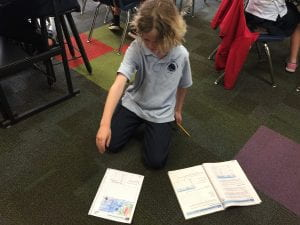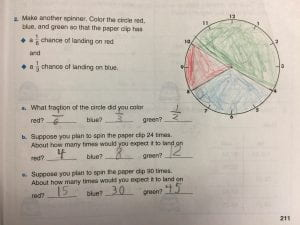## Mrs. Brown's Fourth Grade Blog

### What Are the Odds?

April11

The fourth graders have been learning about probability. As a way to demonstrate this concept, they experimented by dropping a centimeter cube onto a mat that had been shaded with colors in different proportions. They dropped the cube fifty times and recorded their results. We talked about the difference between a guaranteed outcome and a probable outcome.Next we learned that we could calculate the probability of outcomes. Below is a sample from one of their math journals. In this case, students were asked to shade a spinner with three different colors. They wrote down what fraction of the circle was shaded with each color. Based on the fraction, they were able to calculate the probability of landing on each color by asking the question, “What is (fill in with a fraction) of 24 (the number of times we would spin the spinner).